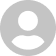Equation Maker
1.2.4

5.0# Equation Maker

1.2.4
22 January 2017

Typeset resolution-independent mathematics.

## Overview

Equation Maker lets you typeset resolution-independent math expressions & equations using LaTeX markup. Edit equations. Export to a PDF or PNG, or drag and drop to another app (Pages, Word, Numbers, Keynote, iBooks Author, PowerPoint, etc).

##### Features:
• Equation updates as you type
• Drag-and-drop as PDF or background transparent PNG
• Syntax and bracket highlighting
• Supports a large set of LaTeX commands
• Palette to recall common LaTeX commands
• Save your equations for later use
• Drag PDFs created with Equation Maker back into Equation Maker to restore equation
• Basic error-reporting

Note: to drag PDF equations from Pages back to Equation Maker: select the PDF image in the Pages document and then on the right select Image tab and drag the small PDF icon from Pages to Equation Maker (the rendering area).

## What's new in Equation Maker

Version 1.2.4:
• Bug fixes (extra spacing bottom of equation in exports)

#### Join over 500,000 subscribers.

Subscribe for our newsletter with best Mac offers from MacUpdate.

## 1 Equation Maker Reviews

Rate this app:13 June 2014